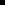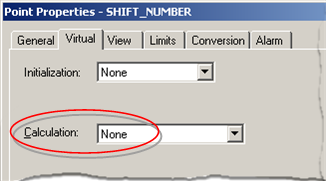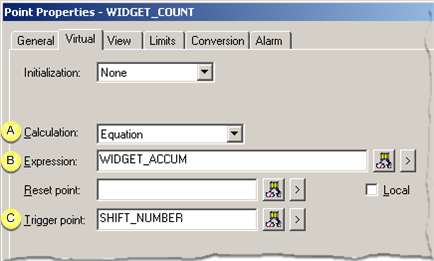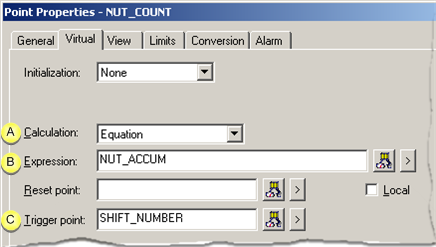#### Examples: Virtual Calculation and Trigger Points

The trigger point function lets you configure virtual points so they are updated only when the trigger point is updated. This minimizes the system resources that are required for calculating virtual points when it is not necessary to keep virtual points current with the points used to calculate their values.

For virtual points, a trigger point may be either a device point or a virtual point.

If the trigger point is a:

 Device point The Update Criteria for the trigger point determines when triggered reads take place. The criteria can either be when the trigger point value changes or when it is scanned. Virtual point There is no update criteria that can be changed.

 Example 1: Virtual Update Logging. Example 2: Virtual Point Interval Trigger.

Example 1: Virtual Update Logging

You are configuring a point that requires an update for logging purposes.

You can use the following based on when the update needs to occur.

 If the update needs to occur: Use Hourly A device trigger point that is configured with a one-hour scan rate. When a particular condition changes: Use either a:Device trigger point that represents the condition and is configured to be updated On Change or.Virtual trigger point that represents the condition.

Example 2: Virtual Point Interval Trigger

Two device points accumulate the number of widgets and nuts made during a shift.WIDGET_ACCUM andNUT_ACCUM

Data needs to be captured from these points at the end of each shift.

A procedure to do this includes configuring three virtual points:SHIFT_NUMBERWIDGET_COUNTNUT_COUNT

1. Configure SHIFT_NUMBER.

1. Select the Virtual tab in the Point Properties dialog box.

2. Select None in the Calculation field.1. Configure WIDGET_COUNT.

Select the Virtual tab in the Point Properties dialog box.

Entries are as follows.Field Description A Calculation Equation B Expression WIDGET_ACCUM The device point that accumulates the number of widgets during the shift. C Trigger point SHIFT_NUMBER When SHIFT_NUMBER changes, WIDGET_COUNT will read the value of WIDGET_ACCUM.
1. Configure NUT_COUNT.

Select the Virtual tab in the Point Properties dialog box.

Entries are as follows.Field Description A Calculation Equation B Expression NUT_ACCUM The device point that accumulates the number of widgets during the shift. C Trigger point SHIFT_NUMBER When SHIFT_NUMBER changes, NUT_COUNT will read the value of NUT_ACCUM.
 Step 3.2. Configure virtual calculations.